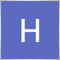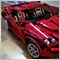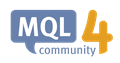# Draw trend line with end122

Hi, from x y point in a bar a line starts and goes to the right if it touches a bar make it stop if not let it continue.

I'm trying to figure out the above but couldn't figure out the code.1218

Hgu Gh:
Hi, from x y point in a bar a line starts and goes to the right if it touches a bar make it stop if not let it continue.

I'm trying to figure out the above but couldn't figure out the code.

You'll need two points, or one point and a slope, to draw a line - do you have these?

Once you have these, nothing stops you from checking bar by bar and compare the price range of that bar against the price value of your line.

Functions you can make use of are: ObjectCreate() and ObjectGetValueByShift(). Once you find the bar that the line touches, simply change the second point of that line to that bar/price, using ObjectSetDouble() to change the y value, and ObjectSetInteger() to change the x value.ObjectGetValueByShift - Object Functions - MQL4 Reference
• docs.mql4.com
The function calculates and returns the price value for the specified bar (shift related to the current bar). The price value is calculated by the first and second coordinates using a linear equation. Applied to trendlines and similar objects. To get the detailed error information, one has to call the GetLastError() function. When this function...122

Seng Joo Thio:

You'll need two points, or one point and a slope, to draw a line - do you have these?

Once you have these, nothing stops you from checking bar by bar and compare the price range of that bar against the price value of your line.

Functions you can make use of are: ObjectCreate() and ObjectGetValueByShift(). Once you find the bar that the line touches, simply change the second point of that line to that bar/price, using ObjectSetDouble() to change the y value, and ObjectSetInteger() to change the x value.

I created a cycle that generates trendlines horizontally when a condition is met and when these horizontal trendlines touch a bar I want it to stop there and if it's not touching anything to go till the end. I still can't see how I can do it with the functions you recommended.

Thanks so muchModerator
20571

Hgu Gh:
I created a cycle that generates trendlines horizontally when a condition is met and when these horizontal trendlines touch a bar I want it to stop there and if it's not touching anything to go till the end. I still can't see how I can do it with the functions you recommended.

Thanks so much

Simply check if the high of a bar >= to the horizontal line && the low of the bar <= the line.

If so, it must have touched, so end the line there.1218

Hgu Gh:
I created a cycle that generates trendlines horizontally when a condition is met and when these horizontal trendlines touch a bar I want it to stop there and if it's not touching anything to go till the end. I still can't see how I can do it with the functions you recommended.

Thanks so much

Well, even simpler if your lines are horizontal, don't even need to use ObjectGetValueByShift()... You want something like these red lines?

See if you can figure out how to do it from these codes (which can go into OnCalculate() or OnTick()):

```   static datetime iLastTime = 0;
datetime iCurrTime = iTime(Symbol(),Period(),1);

for (int i=-10; i<10; i++)
{
if (i==0)
continue;

double dPrice;
string strName = "Line "+string(i);
if (iLastTime==0)
{
datetime iStartTime = iTime(Symbol(),Period(),2);
if (i>0)
dPrice = MathMax(iHigh(Symbol(),Period(),1), iHigh(Symbol(),Period(),2));
else
dPrice = MathMin(iLow(Symbol(),Period(),1), iLow(Symbol(),Period(),2));
dPrice+=(i*Point*20);
ObjectCreate(ChartID(),strName,OBJ_TREND,0,iStartTime,dPrice,iCurrTime,dPrice);
ObjectSetInteger(ChartID(),strName,OBJPROP_RAY,true);
}
else
if (iLastTime<iCurrTime)
{
dPrice = ObjectGetDouble(ChartID(),strName,OBJPROP_PRICE1);
if (dPrice>iLow(Symbol(),Period(),1) && dPrice<iHigh(Symbol(),Period(),1) &&
ObjectGetInteger(ChartID(),strName,OBJPROP_RAY))
{
ObjectSetInteger(ChartID(),strName,OBJPROP_TIME2,iCurrTime);
ObjectSetInteger(ChartID(),strName,OBJPROP_RAY,false);
}
}
}

iLastTime = iCurrTime;
```122

Keith Watford:

Simply check if the high of a bar >= to the horizontal line && the low of the bar <= the line.

If so, it must have touched, so end the line there.

This! Thank you!122

Seng Joo Thio:

Well, even simpler if your lines are horizontal, don't even need to use ObjectGetValueByShift()... You want something like these red lines?

See if you can figure out how to do it from these codes (which can go into OnCalculate() or OnTick()):

Thank you too!122

Keith Watford:

Simply check if the high of a bar >= to the horizontal line && the low of the bar <= the line.

If so, it must have touched, so end the line there.

What im doing wrong? The horizontal lines dont stop where they suppose to stop

```for(int a= 0;a<Bars;a++)
{
string b = IntegerToString(a);
if(1 != 2)
{
ObjectCreate(0,rz+b,OBJ_TREND,0,Time[a],Low[a],Time,Low[a]);
ObjectSetInteger(0,rz+b,OBJPROP_SELECTABLE,0);
ObjectSetInteger(0,rz+b,OBJPROP_RAY_RIGHT,0);
}
double p1= ObjectGetDouble(0,rz+b,OBJPROP_PRICE1);
if(p1 >= Low[a] && ObjectGet(rz+b,OBJPROP_TIME1) < Time[a] && p1 <= High[a])
{
ObjectSet(rz+b,OBJPROP_PRICE2,OBJPROP_PRICE1);
ObjectSet(rz+b,OBJPROP_TIME2,Time[a]);
}
}```Moderator
20571

Hgu Gh:

What im doing wrong? The horizontal lines dont stop where they suppose to stop

`if(1 != 2)`

What is the point of this?

`ObjectCreate(0,rz+b,OBJ_TREND,0,Time[a],Low[a],Time,Low[a]);`

Where is rz declared and given a value?

Why are you trying to create so many objects  in the loop?

I suggest that you decide where and why you want to draw your line. There seems to be no point in just drawing so many lines at the low of each bar at your stage in coding.

Start with a single line and we can take it from there.122

Keith Watford:

What is the point of this?

Where is rz declared and given a value?

Why are you trying to create so many objects  in the loop?

I suggest that you decide where and why you want to draw your line. There seems to be no point in just drawing so many lines at the low of each bar at your stage in coding.

Start with a single line and we can take it from there.

I dont know what im doing wrong because the code looks right but it doesnt gives the desired results...

```   string rz= "RZ";
int a = 42;
string b = IntegerToString(a);
ObjectCreate(0,rz+b,OBJ_TREND,0,Time[a],Low[a],Time,Low[a]);
ObjectSetInteger(0,rz+b,OBJPROP_RAY_RIGHT,0);
double p1= ObjectGetDouble(0,rz+b,OBJPROP_PRICE1);
for(int c= 0; c<Bars;c++)

if(p1 >= Low[c] && iBarShift(0,0,ObjectGet(rz+b,OBJPROP_TIME1),true) > c && p1 <= High[c])
{
ObjectSet(rz+b,OBJPROP_PRICE2,OBJPROP_PRICE1);
ObjectSet(rz+b,OBJPROP_TIME2,Time[c]);
}```1218

Hgu Gh:

I dont know what im doing wrong because the code looks right but it doesnt gives the desired results...

This line:

`ObjectSet(rz+b,OBJPROP_PRICE2,OBJPROP_PRICE1);`

should be:

`ObjectSet(rz+b,OBJPROP_PRICE2,p1);`

But in fact this entire line is not necessary.

`for(int c= 0; c<Bars;c++)`

You could have:

`for(int c=1; c<a;c++)`

So that your:

`if(p1 >= Low[c] && iBarShift(0,0,ObjectGet(rz+b,OBJPROP_TIME1),true) > c && p1 <= High[c])`

could be simplified into:

`if(p1 >= Low[c] && p1 <= High[c])`